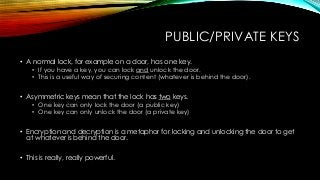Successfully reported this slideshow.

# Public Key Cryptography - A Brief Overview×

1 of 8 Ad
1 of 8 Ad

# Public Key Cryptography - A Brief Overview

A brief overview to public key cryptography. Why it's important, how it works and an example.

A brief overview to public key cryptography. Why it's important, how it works and an example.

## More Related Content

### Public Key Cryptography - A Brief Overview

1. 1. PUBLIC KEY CRYPTOGRAPHY A brief overview By Andy Brodie
2. 2. PUBLIC/PRIVATE KEYS • A normal lock, for example on a door, has one key. • If you have a key, you can lock and unlock the door. • This is a useful way of securing content (whatever is behind the door). • Asymmetric keys mean that the lock has two keys. • One key can only lock the door (a public key) • One key can only unlock the door (a private key) • Encryption and decryption is a metaphor for locking and unlocking the door to get at whatever is behind the door. • This is really, really powerful.
3. 3. BENEFITS OF ASYMMETRIC KEYS • If you give away the public key (that encrypts) then anyone can protect content. • No-one else can decrypt and read the content unless they have the private key. • But, there’s more… it works the other way around too!
4. 4. BUT HOW DO THEY WORK? • Private keys must be unguessable. • It must be impossible to derive the private key from the public key. • Keys are calculated from very, very large prime numbers. • Key “strength” is measure in bits, i.e. the number of bits the key consists of. • E.g. an 8-bit key has a range of 256 potential values (28). • This is not very secure as it would be trivial to manufacture all 256 keys and try them against the lock (a brute force attack) • A 2048 bit key has a range of 3.23x10616. • 3.23 with 616 zeros after the end. • Even the Milliard Gargantubrain could only manage ~1x1057 in a millisecond!
5. 5. MATHS • Select 2 large primes: 𝑝 and 𝑞. • Calculate product of the keys 𝑛 = 𝑝𝑞 • Calculate the totient of 𝑛. • For any integer, 𝑥, the totient of 𝑥, written 𝜑(𝑥) is the number of integers smaller than 𝑥 that are relatively prime to 𝑥. • For any prime number 𝜑 𝑥 = 𝑥 − 1, therefore for the product of two primes, 𝜑(𝑛) = (𝑝 − 1)(𝑞 − 1). • Choose any integer, 𝑒, smaller than and relatively prime to 𝜑(𝑛). 𝑒 is called the public key exponent. • Calculate an integer 𝑑 such that 𝑑𝑒 = 1 𝑚𝑜𝑑 𝜑 𝑛 . • I.e. 𝑑𝑒 𝜑 𝑛 results in any number with remainder 1. • This is done reliably using a multiplicative inverse function… which is TMI for now. • Once calculated, 𝑑 is called the private key exponent. • The public key pair is the modulus and the public key exponent: 𝑛, 𝑒 • The private key is the modulus and the private key exponent: (𝑛, 𝑑)
6. 6. REAL MATHS! • Let’s pick 2 random small primes: 𝑝 = 3, 𝑞 = 11 • Modulus of keys 𝑛 = 𝑝𝑞 = 33 • Totient 𝜑 𝑛 = 3 − 1 11 − 1 = 2 ∗ 10 = 20 • Choose 𝑒, any prime less than 20. Choices are 7, 11, 13, 17, 19. Pick 𝑒 = 7. • Therefore public key pair = (33, 7) • For private key exponent, calculate multiplicative inverse, i.e. 𝑑𝑒 ≡ 𝑚𝑜𝑑 𝜑(𝑛). • 𝑑 ∗ 7 = 1 𝑚𝑜𝑑 20 • I.e. some number, multiplied by 7 and divided by 20 leaves a remainder of 1. • In our heads, we know that 21 𝑚𝑜𝑑 20 = 1, so 𝑑 = 21 7 = 3 • Private key pair = (33, 3)
7. 7. ENCRYPTING AND DECRYPTING • Encrypting and decrypting is (comparatively) very, very simple. • Our key pairs: Public 𝑛, 𝑒 = 33, 7 ; Private 𝑛, 𝑑 = 33, 3 • So, let’s encrypt the number 14 • To encrypt: 𝑝 𝑒 = 𝑝′ 𝑚𝑜𝑑 𝑛 • 𝑝 is the byte to encrypt • 𝑝’ is the encrypted byte • 𝑛 is the modulus • 𝑒 is the public key exponent • Real values: • 147 = 105413504 • 𝑝’ = 10541348 𝑚𝑜𝑑 33 = 𝟐𝟎 • To decrypt: 𝑝′ 𝑑 = 𝑝′ 𝑚𝑜𝑑 𝑛 • 𝑝 is the byte to encrypt • 𝑝’ is the encrypted byte • 𝑛 is the modulus • 𝑒 is the public key exponent • Real values: • 𝟐𝟎3 = 8000 • 𝑝 = 8000 𝑚𝑜𝑑 33 = 𝟏𝟒